How to Learn in 24 Hours?The Rapid Learning Movie

 Need Help? M-F: 9am-5pm(PST): Toll-Free: (877) RAPID-10 US Direct: (714) 692-2900 Int'l: 001-714-692-2900 24/7 Online Technical Support: The Rapid Support Center Secure Online Order:Need Proof? Testimonials by Our Users

 Rapid Learning Courses: MCAT in 24 Hours (2015-16) USMLE in 24 Hours (Boards) Chemistry in 24 Hours Biology in 24 Hours Physics in 24 Hours Mathematics in 24 Hours Psychology in 24 Hours SAT in 24 Hours ACT in 24 Hours AP in 24 Hours CLEP in 24 Hours DAT in 24 Hours (Dental) OAT in 24 Hours (Optometry) PCAT in 24 Hours (Pharmacy) Nursing Entrance Exams Certification in 24 Hours eBook - Survival Kits Audiobooks (MP3)

 Tell-A-Friend: Have friends taking science and math courses too? Tell them about our rapid learning system.Home »  Mathematics »  Pre-Calculus

Exponential Functions, Graphs and Models

 Topic Review on "Title": Domain of f(x): The domain of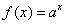consists of all real numbers. Range of f(x): The range ofis the collection of all positive real numbers.   Properties of exponentials:  1)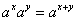2)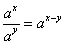3)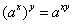4)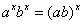Increasing exponential function: If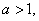then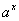is an increasing function. Decreasing function: Ifthenis a decreasing function.

Rapid Study Kit for "Title":
 Flash Movie Flash Game Flash Card Core Concept Tutorial Problem Solving Drill Review Cheat Sheet"Title" Tutorial Summary : Exponential functions are used in mathematics application problems. The operations of exponential functions are defined and used in the examples from this tutorial. Understanding how to plot the graph of an exponential function is important to the discussion of its properties. Euler’s number known as “e” is a famous one that is commonly used in advanced mathematics course and physical science disciplines. Sample problems of the use of the basic exponential function are shown through the examples.

 Tutorial Features: Specific Tutorial Features: • Step by step examples are shown to describe the properties and operations of exponentials. • Solving exponential functions are presented with the use of problem solving techniques. Series Features: • Concept map showing inter-connections of new concepts in this tutorial and those previously introduced. • Definition slides introduce terms as they are needed. • Visual representation of concepts • Animated examples—worked out step by step • A concise summary is given at the conclusion of the tutorial.

 "Title" Topic List: Definition of exponential functions Properties of exponential functions Graph of exponential functions Euler’s number Sample Problems of exponential functions

See all 24 lessons in Pre-Calculus, including concept tutorials, problem drills and cheat sheets:
Teach Yourself Pre-Calculus Visually in 24 Hours